Using Decimals - Converting to mm, cm, km, kg, rupees

Chapter 8 Class 6 Decimals
Concept wise

Converting mm to cm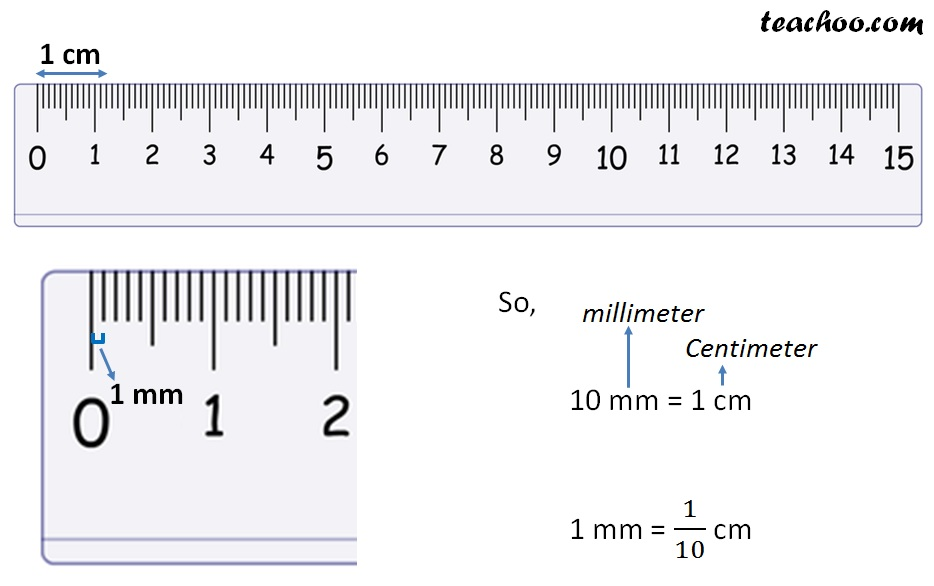### Convert 4 mm in cm

4 mm = 4 × 1 mm

= 4 × 1/10 cm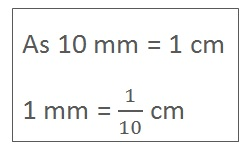= 4/10 cm

= 04/10 cm

= 0.4 cm

∴ 4mm = 0.4 cm

### Convert 245 mm in cm

245 mm = 245 × 1 mm

= 245 × 1/10 cm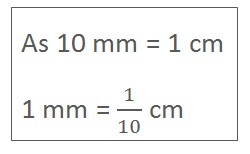= 245/10 cm

= 24.5 cm

∴ 245mm = 24.5 cm

### Converting cm to m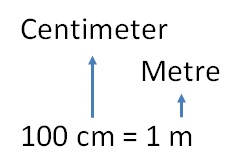1Cm = 1/100 m

Convert 29 cm into m

29 cm = 29 × 1 cm

= 29 × 1/100 m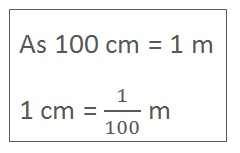= 29/100 m

= 029/100 m

= 0.29 m

### Convert 186 cm into m

186 cm = 186 × 1 cm

= 186 × 1/100 m

= 186/100 m

= 1.86 m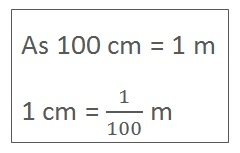### Converting m to km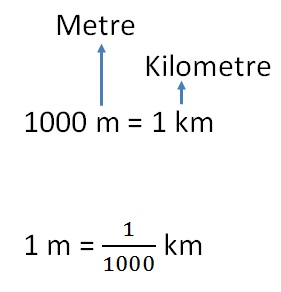### Convert 19 m into km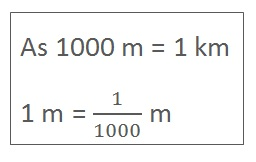19 cm = 19 × 1 m

= 19 × 1/1000 km

= 19/1000 km

= 0019/1000 km

= 0.019 km

### Convert 1899 m into km

1899 m = 1899 × 1 m

= 1899 × 1/1000 km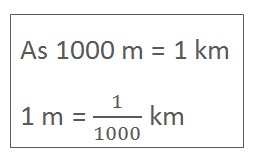= 1899/1000 km

= 1.899 km

Learn in your speed, with individual attention - Teachoo Maths 1-on-1 Class

### Transcript

Converting to mm, cm, m, kmConverting mm to cm Convert 4 mm in cm 4 mm = 4 × 1 mm = 4 × 1/10 cm = 4/10 cm = 04/10 cm = 0.4 cm ∴ 4mm = 0.4 cm Convert 4 mm in cm 4 mm = 4 × 1 mm = 4 × 1/10 cm = 4/10 cm = 04/10 cm = 0.4 cm ∴ 4mm = 0.4 cm Convert 4 mm in cm 4 mm = 4 × 1 mm = 4 × 1/10 cm = 4/10 cm = 04/10 cm = 0.4 cm ∴ 4mm = 0.4 cm Convert 245 mm in cm 245 mm = 245 × 1 mm = 245 × 1/10 cm = 245/10 cm = 24.5 cm ∴ 245mm = 24.5 cm 100 cm = 1 m 1 cm = 1/100 m Convert 29 cm into m 29 cm = 29 × 1 cm = 29 × 1/100 m = 29/100 m = 029/100 m = 0.29 m Convert 186 cm into m 186 cm = 186 × 1 cm = 186 × 1/100 m = 186/100 m = 1.86 m 1000 m = 1 km 1 m = 1/1000 km Convert 19 m into km 19 cm = 19 × 1 m = 19 × 1/1000 km = 19/1000 km = 0019/1000 km = 0.019 km Convert 1899 m into km 1899 m = 1899 × 1 m = 1899 × 1/1000 km = 1899/1000 km = 1.899 km We know that 100 paise = ₹ 1 1 paise = ₹ 1/100 Convert 86 paise into rupees 86 paise = 86 × 1 paise = 86 × 1/100 rupees = (86 )/100 rupees = 086/100 = 0.86 rupees Convert 123 paise into rupees 123 paise = 123 × 1 paise = 123 × 1/100 rupees = 123/100 rupees = 1.23 rupees 1000 g = 1 kg 1 g = 1/1000 kg Convert 28 g into kg 28 g = 28 × 1 g = 28 × 1/1000 kg = 28/1000 kg = 0028/1000 = 0.028 kg